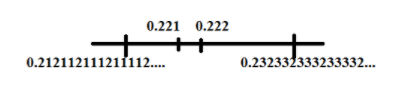Courses
Courses for Kids
Free study material
Free LIVE classes
MoreLIVE
Join Vedantu’s FREE Mastercalss

# Give two rational numbers lying between 0.232332333233332….. and 0.212112111211112…..Enter 1 if the answer is 0.221, 0.222 otherwise enter 0.Verified
334.5k+ views
Hint: In this particular question use the concept that rational number are terminating and recurring decimals whereas irrational numbers are non-terminating and non-recurring decimals and between two numbers there are infinitely many numbers are possible so use these concepts to reach the solution of the question.

Given numbers
0.232332333233332….. and 0.212112111211112…..
As we all know that irrational numbers are non – terminating and non – recurring decimals i.e. it cannot written in the form of $\dfrac{p}{q}$, where $q \ne 0$and p and q has not any common factors except 1.
So the given numbers are irrational numbers so it cannot be written in the form of $\dfrac{p}{q}$, where $q \ne 0$.
Now we have to find out the two rational numbers between the given numbers.
As we all know between these two irrational numbers there are infinitely many numbers possible some are rational numbers and some are irrational numbers.
So 0.221 and 0.222 is greater than 0.212112111211112…. and less than 0.232332333233332…. so rational numbers 0.221 and 0.222 are lying between them as shown in the below number line.Hence 0.221 and 0.222 are lying between the given irrational numbers.
So according to the question we have to enter 1.

Note: Whenever we face such types of questions the key concept we have to remember is that always recall that the rational numbers are written in the form of $\dfrac{p}{q}$, where $q \ne 0$ and irrational numbers cannot written in the form of $\dfrac{p}{q}$, where $q \ne 0$, where p and q has not any common factors except 1.

Last updated date: 21st Sep 2023
Total views: 334.5k
Views today: 6.34k